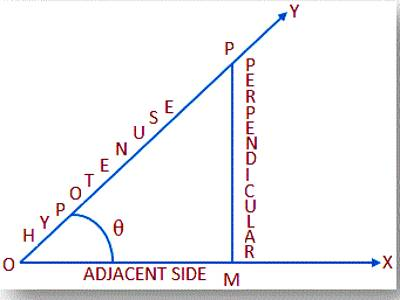Quotient Relations of Trigonometric Ratios

The quotient identities tell us relationships among functions in threes: the tangent function is the quotient of the sine and cosine functions, and the cotangent function is the reciprocal of this quotient. For any right triangle, there are six trig ratios: Sine (sin), cosine (cos), tangent (tan), cosecant (csc), secant (sec), and cotangent (cot). Here are the formulas for these six trig ratios: Given a triangle, you should be able to identify all 6 ratios for all the angles (except the right angle).

The quotient that represents a trigonometric function by the division of a trigonometric function by another trigonometric function is called the quotient relation of trigonometric functions. In trigonometry, a trigonometric function can be divided by another trigonometric function and the quotient of them is also a trigonometric function. Hence, it is called the quotient identity of trigonometric functions.

Quotient relations of trigonometric ratios are tan θ = sin θ/cos θ and cot θ = cos θ/sin θ.

Let OMP be a right-angled triangle at M and ∠MOP = θ.Quotient relation of trigonometric ratios :

• When we divide the trigonometric ratio sinθ by cosθ, the quotient is tanθ.
• When we divide the trigonometric ratio cosθ by sinθ, the quotient is cotθ.
• When we divide the trigonometric ratio cscθ by secθ, the quotient is cotθ.
• When we divide the trigonometric ratio secθ by cscθ, the quotient is tanθ.

The above said divisions are called as quotient relation of trigonometric ratios.

According to the definition of trigonometric ratios we have,

sin θ = perpendicular/hypotenuse = MP/OP ………….. (i)

and cos θ = adjacent/hypotenuse = OM/OP ………….. (ii)

Now dividing (i) by (ii) we get;

sin θ/cos θ = (MP/OP)/(OM/OP)

= (MP/OP) × (OP/OM)

= MP/OM

= tan θ

Therefore, tan θ = sin θ/cos θ

Again dividing (ii) by (i) we get;

cos θ/sin θ = (OM/OP)/(MP/OP)

= (OM/OP) × (OP/MP)

= OM/MP

= cot θ

Therefore, cot θ = cos θ/sin θ

The above step-by-step explanation on quotient relations of trigonometric ratios is very important to solve various problems on trigonometry.

Information Source: